At the end of March, I began to apply R to some subjects. There are lots of traps during the programming. Sometimes, it took me hours only found that the speciality of some structures or function. For example, at first, I thought it would be extremely easy when I tried to plot through ggplot in a loop. However, days after I have implemented a serials of plots, I found all the plots have the same outlook. S3 and s4 object as well as vector, list, dataframe sometimes draw me crazy.

### strange plots

When I tried to plot bars, I found there were some strange guys in the several bar plots. Here is the task: I need plot a list of data, each element will be a bar plot. First of all, I initialize the plot parameters as followed:

fortify is a way of coverting a list to dataframe. To sort log.m, just put order(log.m[,1]) as new idx. Next step is to get top 20 of log.m and then sort the 20 elements.

The descriptions are too long sometimes so that I tried to find a way to cutoff longer strings. That is, to change the log.m\$names = df\$Description line. I searched for substring function and found str_sub from package stringr to finish the job:

The code seems to work well, bar plots are supposed to list in order but they’re not: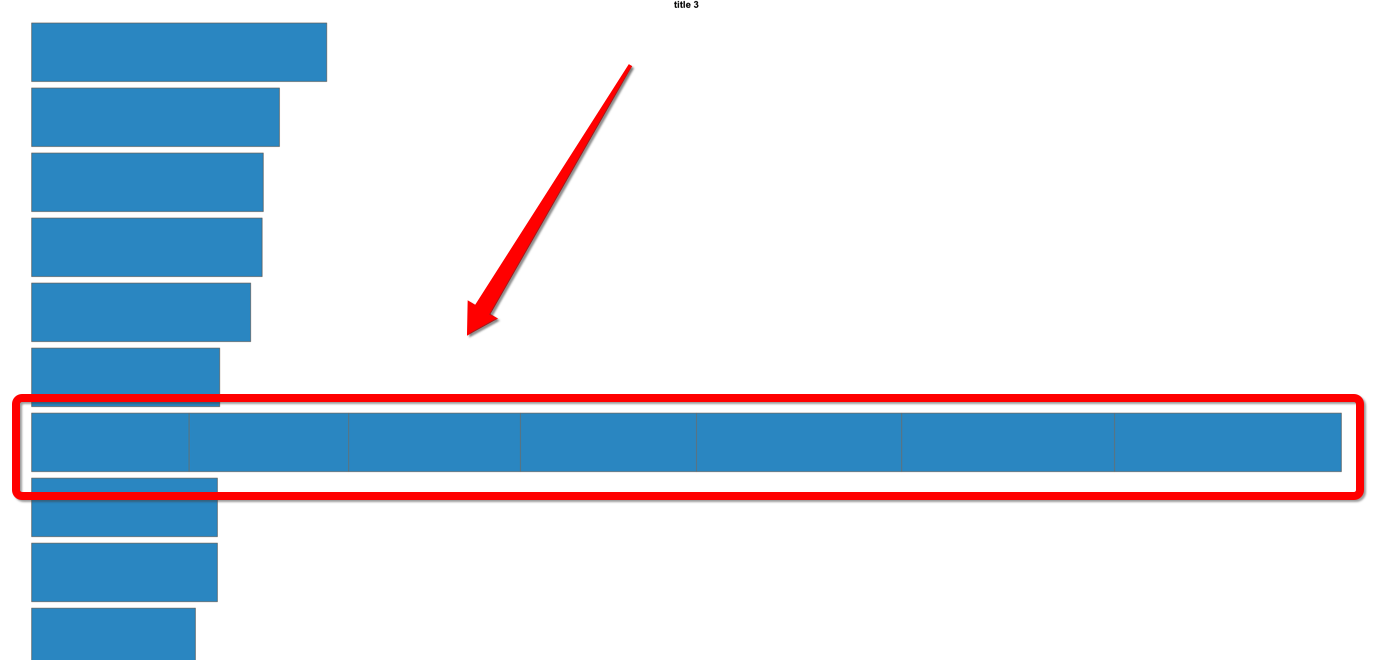To inspect the differences between cutoff and the original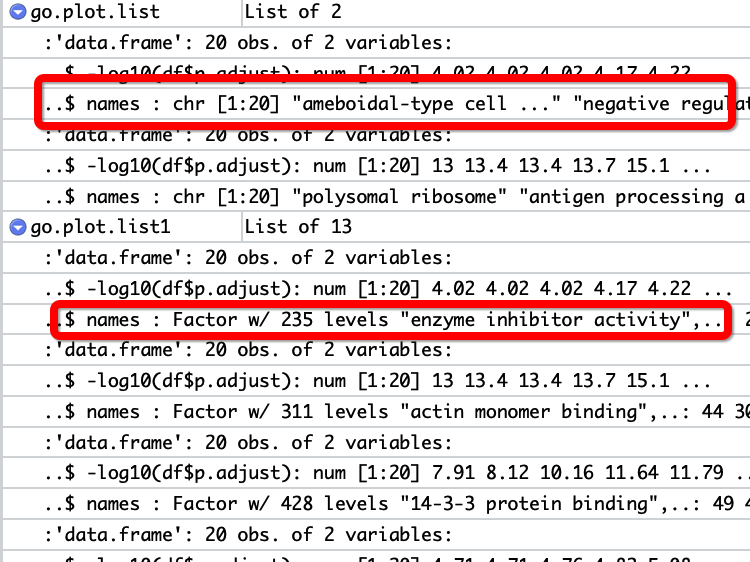Note that, the type of two names are different: the former list is string list whereas the latter one is factor. To keep the same type, I use as.factor() function to convert string list as factor:

It turns out that plots still performance abnormally although the type of names has become as factor. Now, the only differences is the levels for the two list. I just mask the str_sub function to see if the other parts work well or not.

Yeah, the stacked bars on plots disappear now. I should draw the conclusion that it is the function str_sub which lead to the abnormal plots. So the following code should work well as I expected:

But wait. ʕฅ•ω•ฅʔ, when I check the name factor after I changed the code as:

It seems that the shorter kept length of string, the more serious on the plot:When I looked at the plot, I found the label negative r. It seems this negative r can plot for more than one times. Is there a possibility that all the bars with the negative r label are ploted together?. First, let’s look at how many negative r in the factor? 7 times.

Now, for plot.list[], there are 6 joint line which means 7 bars on one line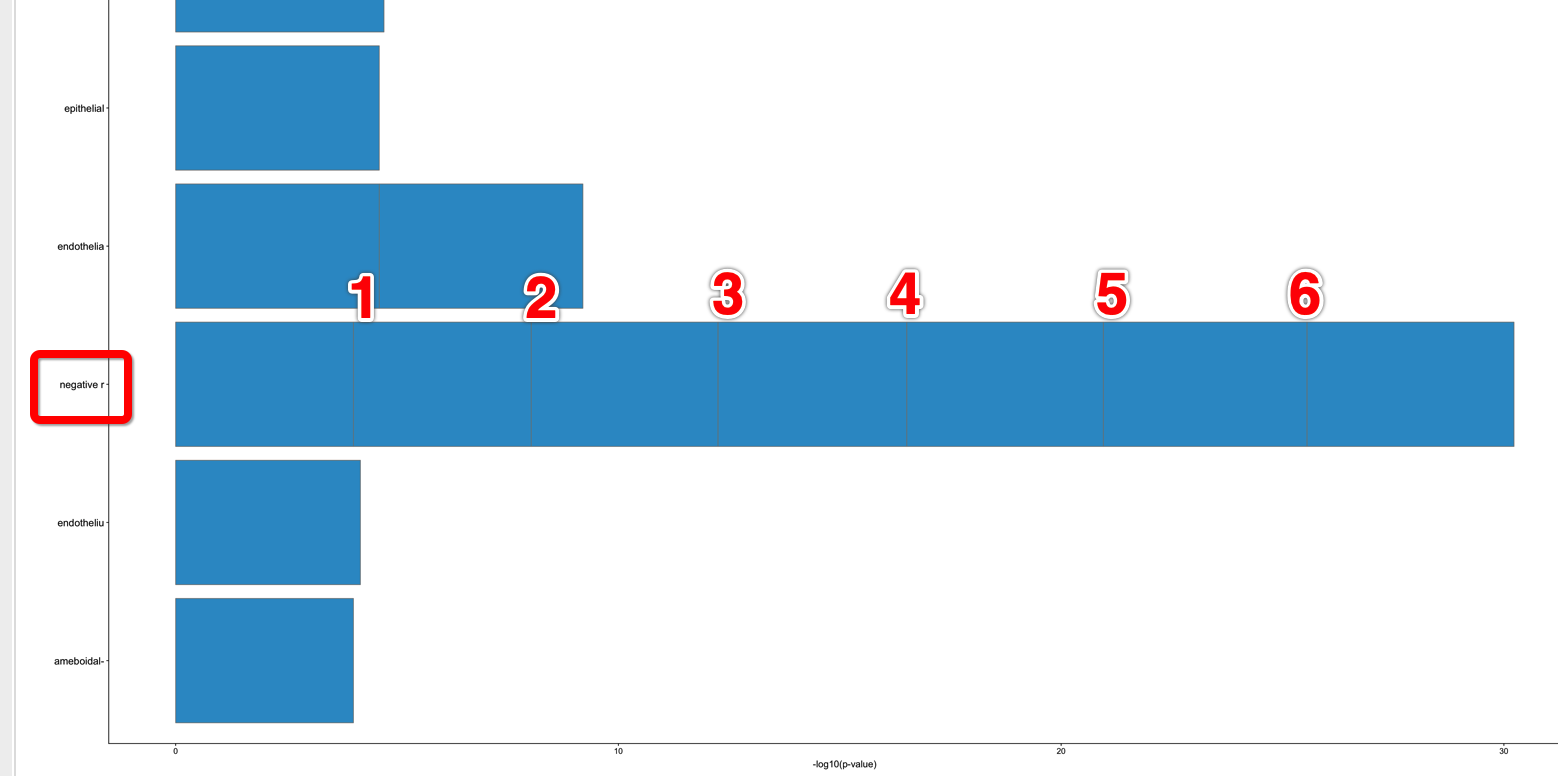See the extreme condition, now we can set only one level for the name factor, that is:

Maybe you can imagine how the plot looks like. All 20 bars stack together in one line.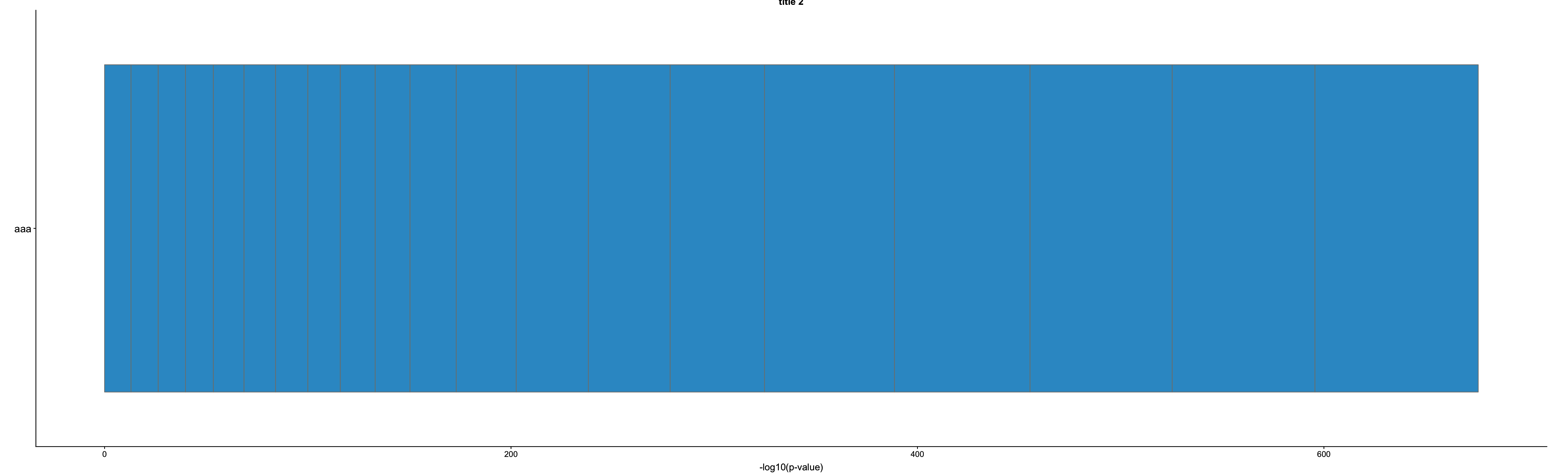If we want to keep shorter string, in the mean time, we still hope not be borthered by the same label problem, we can introduce hash function to solve the problem.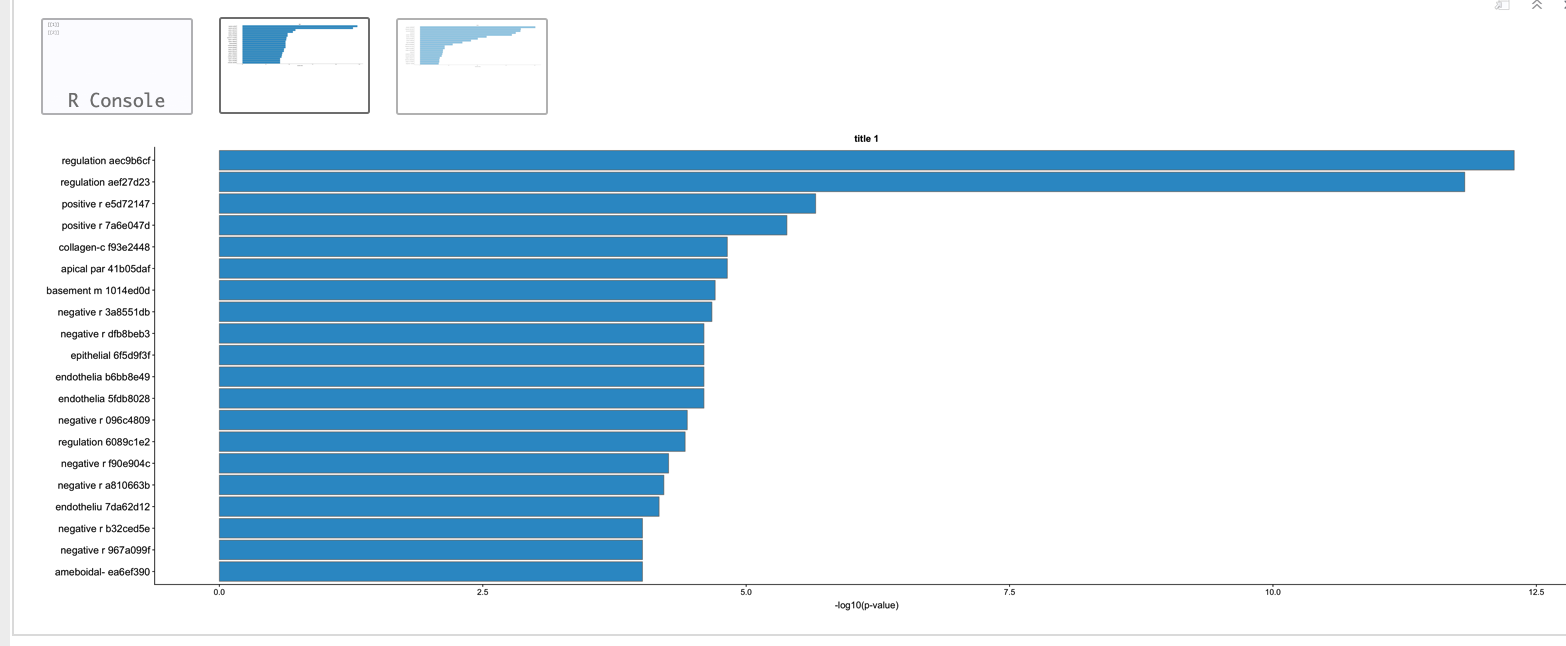The code of plots is as follows

## All same plots

There are too many plots so that it is a good idea to put multiple graphs on one page.

This is what happened, all plots were the same after plot in a loop. At first I thought, I didn’t allocate memory for the up.list because I initialized up.list as followed:

Then, I changed the initialization:

Still, all plots are identical. Code for ploting seems correct:

But from the 4 plots in one page we can see that every plot is the same as the others.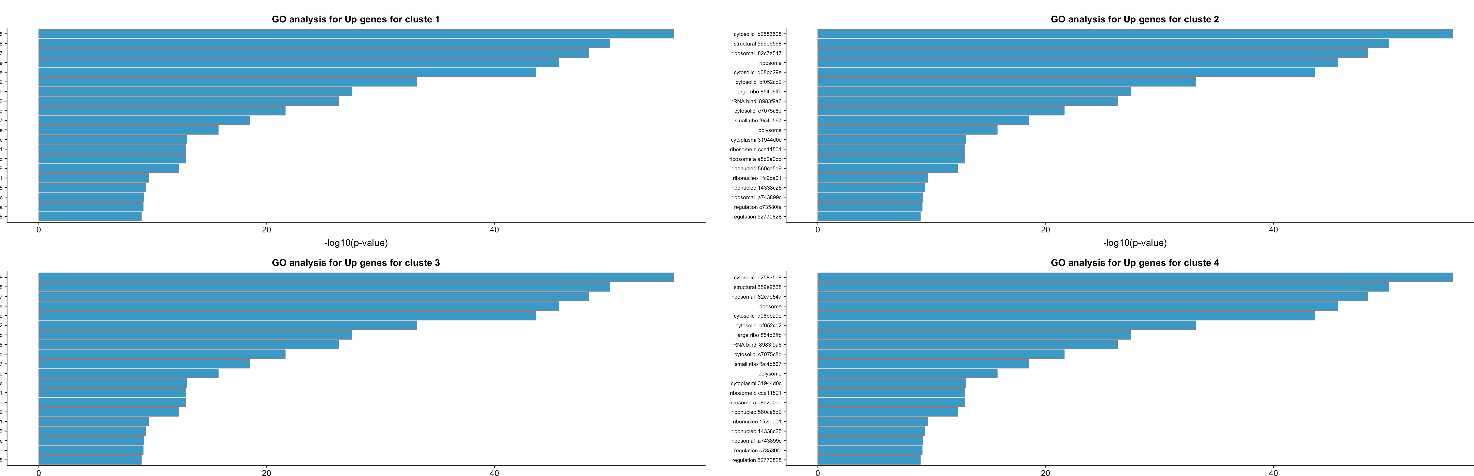For now, I haven’t found solution to this problem.